• 看网上的介绍,对于字符串常量池中到底保存的是字符串对象,还是字符串对象的引用,众说纷纭… 看 jdk1.8 对 intern() 的说明. When the intern method is invoked, if the pool already contains a string equal to ...
前言
这篇文章写的比较烂，是我的一点笔记，当时自己没怎么思考就贴在这儿了…这不误人子弟吗…
推荐各位看美团官方技术团队博客的这篇文章，应该说讲得很清楚详细了。
这里
按我的理解，应该是 jdk7 及以后 字符串常量池 既【可以】保存 String 对象，又【可以】保存 String 对象的引用。
    String s3 = new String("1") + new String("1");
s3.intern();
String s4 = "11";
System.out.println(s3 == s4);

这段代码在 jdk 7 及以后，返回值是 true，便说明了这点。
正文
看网上的介绍,对于字符串常量池中到底保存的是字符串对象,还是字符串对象的引用,众说纷纭…
看 jdk1.8 对 intern() 的说明.

When the intern method is invoked, if the pool already contains a string equal to this String object as determined by the equals(Object)method, then the string from the pool is returned. Otherwise, this String object is added to the pool and a reference to this String object is returned.

这个说明对于理解原理其实没有啥参考价值…至于为啥,往下看…
尝试跟踪源码.
public native String intern();

是个本地方法,继续深入 :
在线查看
\openjdk7\jdk\src\share\native\java\lang\String.c
Java_java_lang_String_intern(JNIEnv *env, jobject this)
{
return JVM_InternString(env, this);
}

日…到这步跟不下去了.还是搜索一下看看别人有没有关于这玩意儿的资料吧…
果然找到!!
深入解析String#intern
详细的看上面吧,这里直接给结果了…
看段代码：
public static void main(String[] args) {
String s = new String("1");
s.intern();
String s2 = "1";
System.out.println(s == s2);

String s3 = new String("1") + new String("1");
s3.intern();
String s4 = "11";
System.out.println(s3 == s4);
}

jdk6 输出 : false false
jdk7 输出 : false true

why?
jdk6 上面已经提到过,String s = new String("1"); 这种方式创建字符串实际生成了两个字符串对象. 首先,构造器中传入了一个字符串对象 “1”,它就被放在字符串常量池中. 然后在堆中创建一个字符串对象,s 指向这个字符串对象的首地址. 调用 s.intern() ,字符串常量池已经存在该对象,直接返回. s2 指向的就是字符串常量池中 “1” 的首地址. 而 == 判断的是地址是否相同.显然不同. s3 与 s4 不同之处在于,执行 s3.intern() 时,字符串常量池中还没有字符串对象,所以需要在字符串常量池中创建一个对象.其余的与上相同.  jdk 7 s 与 s2 情况与 jdk6 一样. 但是执行 s3.intern() 时,字符串常量池中还没有字符串对象,此时先堆中有没有该字符串对象,如果有,就把堆中字符串对象的引用保存到字符串常量池中. 所以 s4 指向的和 s3 一样,都是堆中 String 对象的引用. 所以判断为 true.
总结 :
jdk6 : 当调用 intern 方法时,如果字符串常量池先前已创建出该字符串对象,则返回池中的该字符串的引用.否则,将该字符串对象添加到字符串常量池中,并且返回该字符串对象的引用.
jdk6+ : 当调用 intern 方法时,如果字符串常量池先前已创建出该字符串对象,则返回池中的该字符串的引用.否则,如果该字符串对象已经存在于 Java堆 中,则将堆中对此对象的引用添加到字符串常量池中,并且返回该引用;如果堆中不存在,则在池中创建该字符串并返回其引用.
jdk6 字符串只存在于 字符串常量池.
jdk6+ 字符串存在于字符串常量池和 Java堆.
那么回到上面那个问题,字符串常量池中到底保存的是字符串对象,还是字符串对象的引用?
答案就是,jdk6 保存的是字符串对,jdk6+ 既保存了字符串对象,又保存了字符串对象的引用.
而上面之所以说 jdk 对 intern() 的说明对于理解原理其实没有啥参考价值…就是因为 jdk6 和 jdk 8 的说明是一样的,而它们明明有差别嘛…
展开全文String java
• 字符串对象有三种编码方式 第一种编码方式int 第二种编码方式raw 第三种编码方式embstr 如果字符串对象保存的是一个字符串值，并且这个字符串值的长度小于等于39个字节，那么字符串对象将使用embstr...
字符串对象有三种编码方式

第一种编码方式int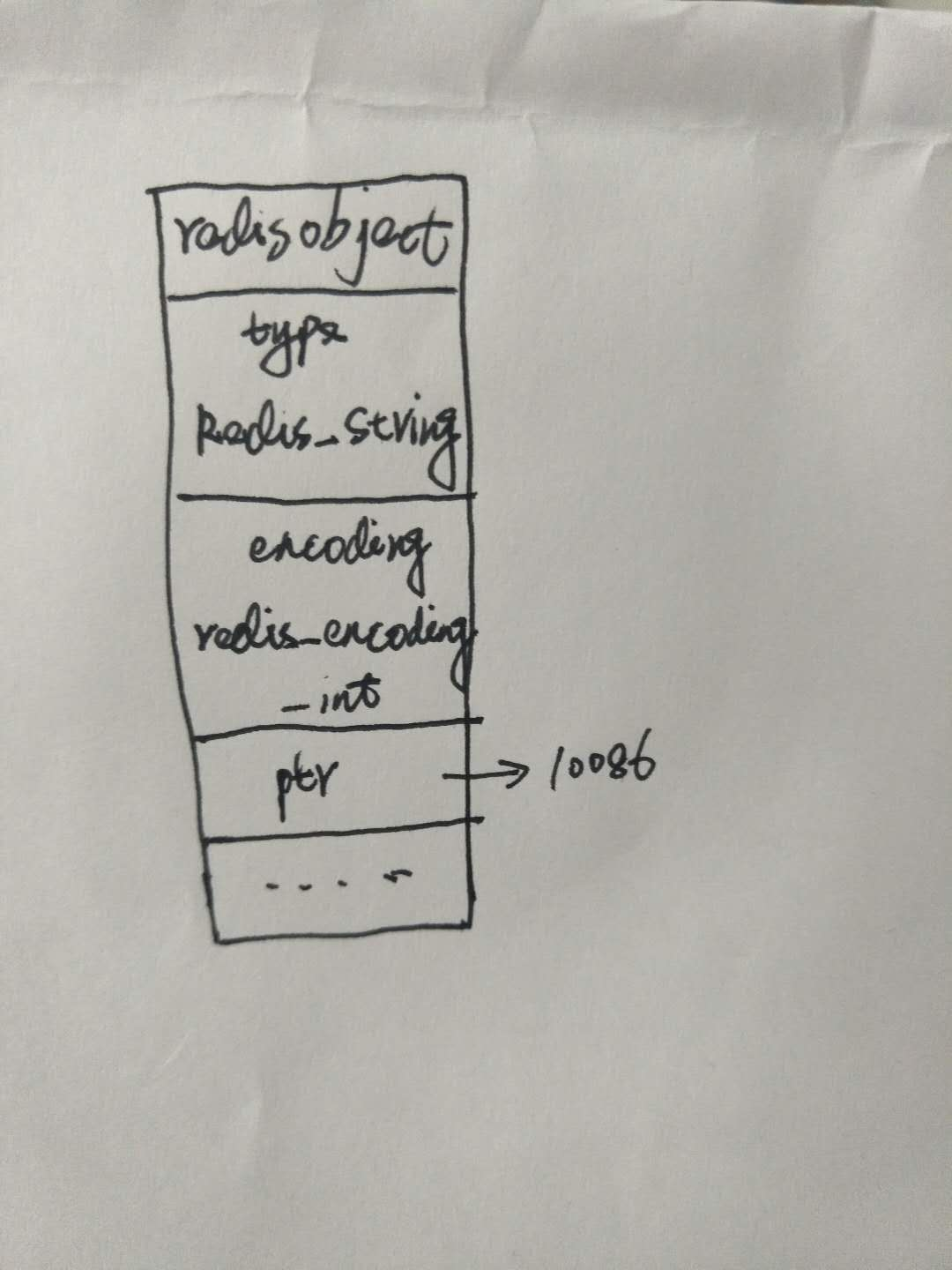第二种编码方式raw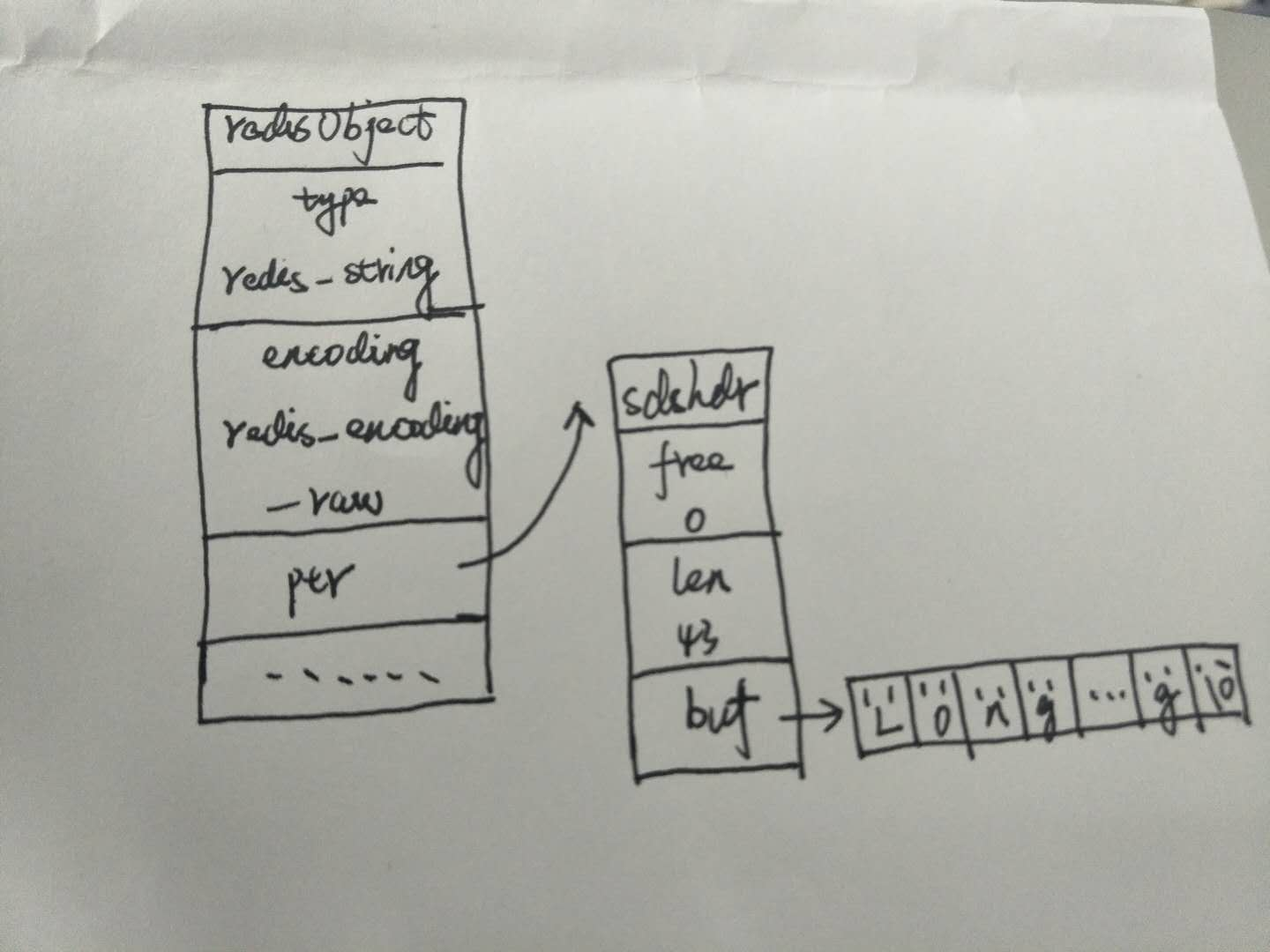第三种编码方式embstr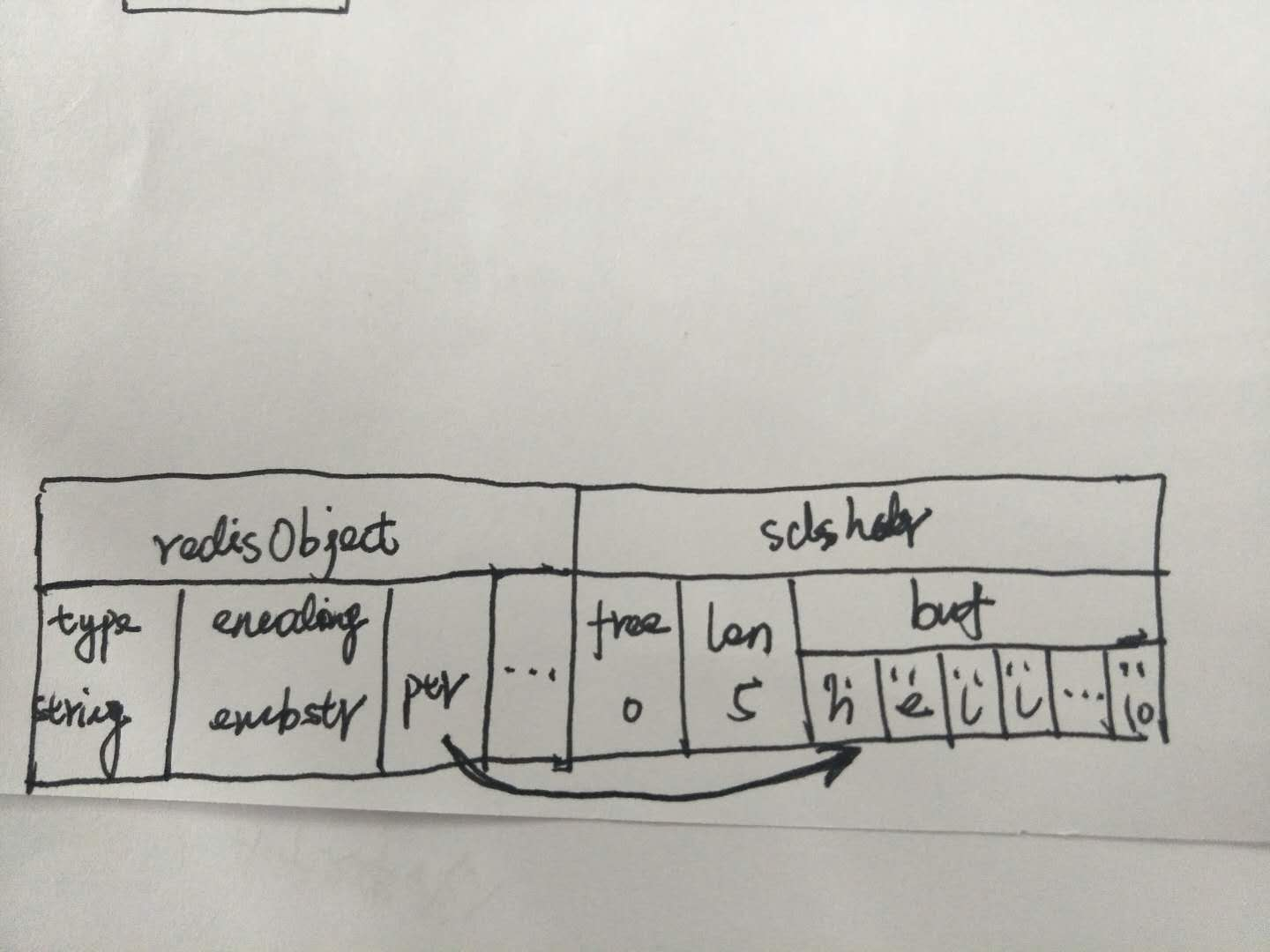如果字符串对象保存的是一个字符串值，并且这个字符串值的长度小于等于39个字节，那么字符串对象将使用embstr编码的方式来保存这个值。
使用embstr编码的字符串对象来保存短字符串值有以下好处：
embstr编码将创建字符串对象所需的内存分配次数从raw编码的二次降低为一次。释放embstr编码的字符串对象只需要调用一次内存释放函数，而释放raw编码的字符串对象需要调用二次内存释放函数。embstr能够更好的利用缓存带来的优势。 另外embstr编码的字符串对象没有对应的修改程序，所以任何embstr编码的字符串对象只要修改，就会自动转换编码为raw的。
展开全文redis
• C++ 字符串 对象 C++ 字符串 对象 创建方法 C++ 字符串 对象 输出到控制台 C++ 字符串 拼接 C++ 字符串 方法调用 ( 栈内存对象 ) C++ 字符串 方法调用 ( 堆内存对象 ) C / C++ 字符串 完整代码示例


文章目录
C++ 字符串 对象C++ 字符串 对象 创建方法C++ 字符串 对象 输出到控制台C++ 字符串 拼接C++ 字符串 方法调用 ( 栈内存对象 )C++ 字符串 方法调用 ( 堆内存对象 )C / C++ 字符串 完整代码示例

C++ 字符串 对象

C++ 字符串 :
1.string 类 : C++ 中的字符串是 string 类 对象 ;2.string 类定义位置 : 该 string 类定义在 iostream 头文件中 , 不必引入另外的头文件 ;
#include <iostream>


C++ 字符串 对象 创建方法

C++ 字符串 对象 创建方法 :
1.直接赋值 : 直接使用 C 字符串赋值 ;
	//① 使用字符串赋值
string string_c_plus_1 = " Hello ";

2.构造方法 : 调用 string 构造方法 创建 string 对象 ;
① 传入 string 参数 : string 构造方法可以传入 string 对象 ;② 传入 C 字符串参数 : string 构造方法也可以传入 一个 C 字符串 ;
	//② 调用构造方法 初始化字符串
string string_c_plus_2(string_c_plus_1);
string string_c_plus_3(" World ");

3.使用 new 分配内存 : 使用 new 为 String 对象分配一块堆内存空间 , 返回 string * 类型的指针 ;
	//③ 使用 new 申请的内存 , 需要使用 delete 释放
string *string_c_plus_4 = new string(" New ");
delete string_c_plus_4;


调用该 string* 对象的方法需要使用 -> 进行调用 ;

堆内存使用原则 : ① 使用 new 申请的内存 , 需要使用 delete 释放 ; ② 使用 new [] 申请的数组 , 需要使用 delete[] 释放 ;  ③ 使用 malloc 申请的内存 , 需要使用 free 释放 ;

C++ 字符串 对象 输出到控制台

C++ 字符串 对象 输出到控制台 :
1.不能直接使用 cout 输出 : 下面的用法是错误的 ; 使用 cout 不能直接将 string 对象输出 ;
	//这种用法是错误的
string string_c_plus_1 = " Hello ";
cout << string_c_plus_1 << endl;

2.输出方法 : 需要将 string 对象转成 C 字符串 , 然后再输出 :
	//调用 string 对象的 c_str() 方法 , 获取其 C 字符串 , 然后再输出到控制台
string string_c_plus_1 = " Hello ";
cout << string_c_plus_2.c_str() << endl;


在 Java 中输出 String 对象 , 默认会调用其 toString 方法 , 但是在 C 中不可以直接使用 ;

C++ 字符串 拼接

C++ 字符串拼接 :
1.使用 “+” 符号拼接 : 这里的加号进行了操作符重载 , 字符串之间相加代表了字符串的拼接操作 ;
	//① "+" : 操作符重载 , 重新定义了 加号运算符的行为 ,
//		这里加号可以实现字符串拼接 , 与 Java 类似
string string_c_plus_5 = string_c_plus_1 + string_c_plus_3;

2.调用 string 对象的 append 方法 : 调用 string 对象的 append() 方法 , 传入要拼接的字符串 ;
	//② 调用 string 对象的 append 方法 , 拼接字符串
string string_c_plus_6 = string_c_plus_1.append( string_c_plus_3 );

3.代码示例 :
	//C++ 字符串拼接
//① "+" : 操作符重载 , 重新定义了 加号运算符的行为 , 这里加号可以实现字符串拼接 , 与 Java 类似
//② 调用 string 对象的 append 方法 , 拼接字符串
string string_c_plus_5 = string_c_plus_1 + string_c_plus_3;
string string_c_plus_6 = string_c_plus_1.append( string_c_plus_3 );

4.运行结果 :
 Hello  World
Hello  World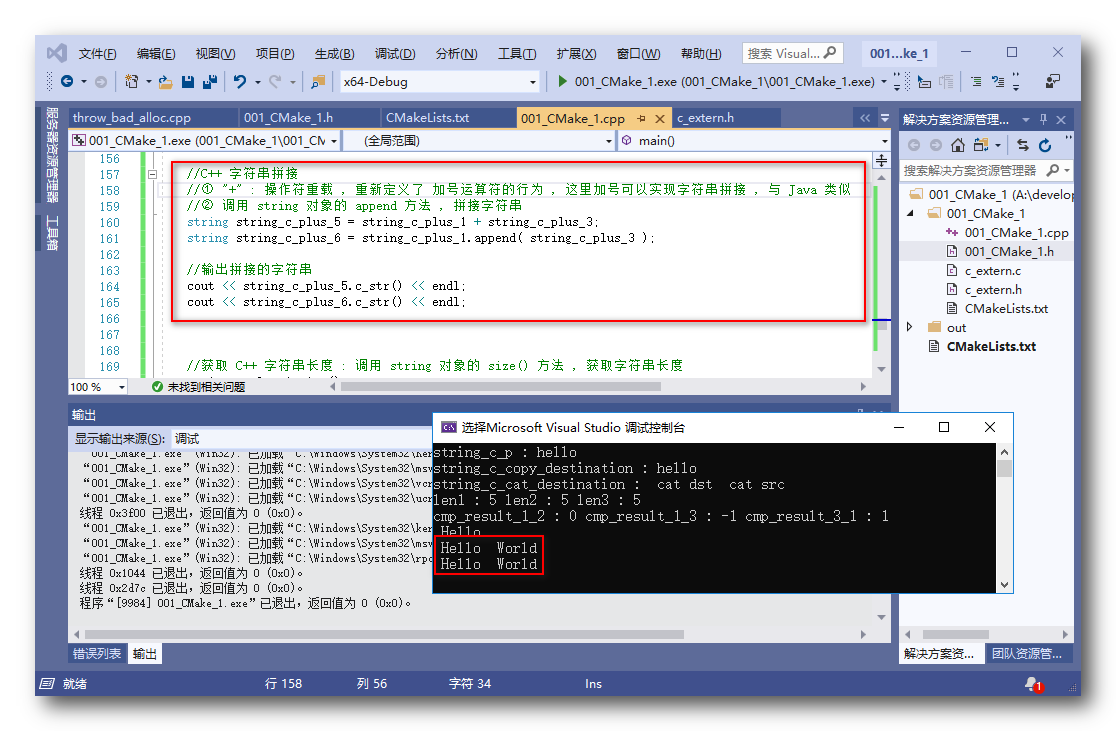操作符重载 : 重新定义了操作符的作用 , 这里的 “+” 就是重新定义了其作用 , 拼接字符串 ;

C++ 字符串 方法调用 ( 栈内存对象 )

C++ 字符串 方法调用 : 该字符串 ( string ) 对象 在栈内存中 ;
1.获取字符串长度 : 调用 string 对象的 size() 方法 , 即可返回字符串长度 ;
	//① 使用字符串赋值
string string_c_plus_1 = " Hello ";
//获取 C++ 字符串长度 : 调用 string 对象的 size() 方法 , 获取字符串长度
int string_c_plus_1_size = string_c_plus_1.size();

2.判断字符串是否为空 : 调用 string 对象的 empty() 方法 , 判断字符串是否为空 ;
	//① 使用字符串赋值
string string_c_plus_1 = " Hello ";
//判断 C++ 字符串是否为空 : 调用 string 对象的 empty() 方法 ;
bool string_c_plus_1_empty = string_c_plus_1.empty();

3.代码示例 :
	//使用字符串赋值
string string_c_plus_1 = " Hello ";

//获取 C++ 字符串长度 : 调用 string 对象的 size() 方法 , 获取字符串长度
int string_c_plus_1_size = string_c_plus_1.size();
cout << "string_c_plus_1_size : " << string_c_plus_1_size << endl;

//判断 C++ 字符串是否为空 : 调用 string 对象的 empty() 方法 ;
bool string_c_plus_1_empty = string_c_plus_1.empty();
cout << "string_c_plus_1_empty : " << string_c_plus_1_empty << endl;

4.运行结果 :
string_c_plus_1_size : 14
string_c_plus_1_empty : 0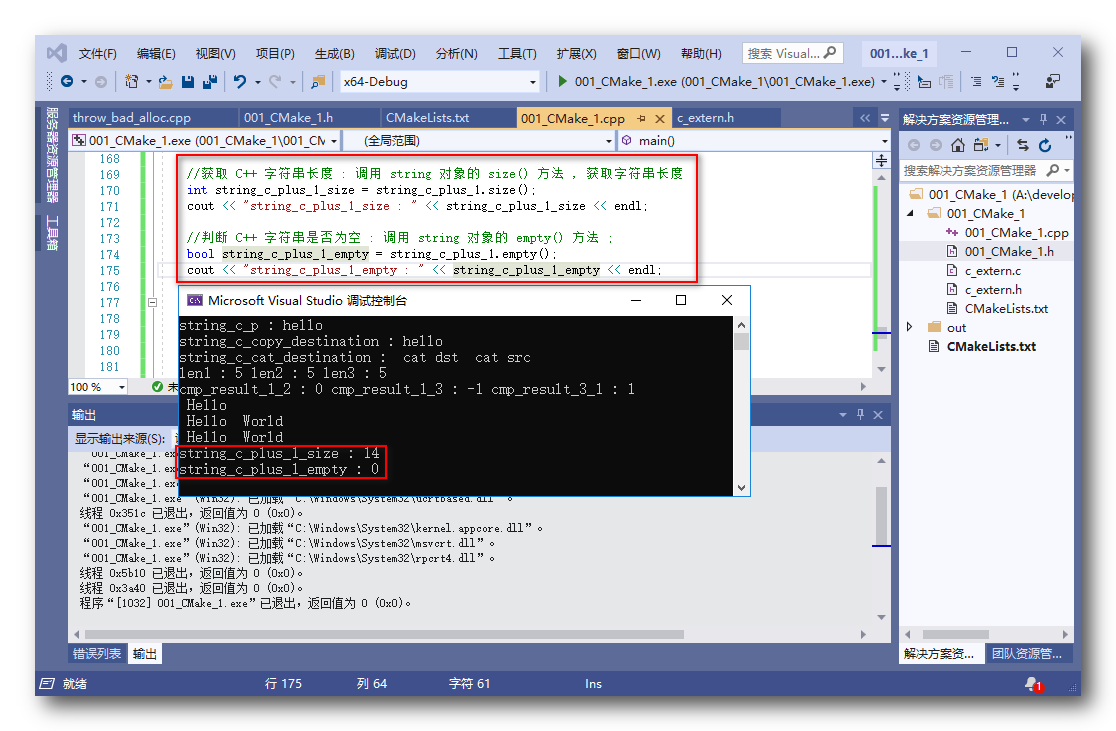使用 “.” 方式调用栈内存对象的方法 ;

C++ 字符串 方法调用 ( 堆内存对象 )

C++ 字符串 堆内存对象 方法调用 :
1.堆内存对象创建 : 使用 new 创建的 string 对象 需要在堆内存中为其分配内存 , 返回的是 string* 指针类型 ;
	//使用 new 创建的对象 , 就不能使用 . 访问其方法和变量 , 需要使用 -> 符号进行访问
// -> 相当于 (*). 运算, 先读取指针内容 , 然后访问其方法或变量

string* string_c_plus_7 = new string(" New String ");

1.获取字符串长度 : 调用 string 对象的 size() 方法 , 即可返回字符串长度 ; 需要使用 -> 代替 . 操作 ;
	//① 获取字符串长度 :
int string_c_plus_7_size = string_c_plus_7->size();

2.判断字符串是否为空 : 调用 string 对象的 empty() 方法 , 判断字符串是否为空 ; 需要使用 -> 代替 . 操作 ;
	//② 判断字符串是否为空 :
bool string_c_plus_7_empty = string_c_plus_7->empty();

3.使用指针的好处 :
① 扩大作用域 : 如果在栈内存中使用 , 有作用域限制 , 出了栈内存 作用域 , 该对象就无效了 ;② 方便参数传递 : 指针 大小为 4 ( 32 位系统 ) 或 8 ( 64 位系统 ) 个字节 , 其当做参数传递 比直接传递对象 ( 动辄几十上百字节甚至更高 ) 效率更高 ; 4.代码示例 :
	//使用 new 创建的对象 , 就不能使用 . 访问其方法和变量 , 需要使用 -> 符号进行访问
// -> 相当于 (*). 运算, 先读取指针内容 , 然后访问其方法或变量

string* string_c_plus_7 = new string(" New String ");

//① 获取字符串长度 :
int string_c_plus_7_size = string_c_plus_7->size();
cout << "string_c_plus_7 : " << string_c_plus_7_size << endl;

//② 判断字符串是否为空 :
bool string_c_plus_7_empty = string_c_plus_7->empty();
cout << "string_c_plus_7_empty : " << string_c_plus_7_empty << endl;

//释放堆内存
delete string_c_plus_7;

//使用指针的好处 :
// ① 如果在栈内存中使用 , 有作用域限制 , 出了栈内存 作用域 , 该对象就无效了 ;
// ② 指针 大小为 4 ( 32 位系统 ) 或 8 ( 64 位系统 ) 个字节 ,
//	  其当做参数传递 比直接传递对象 ( 动辄几十上百字节甚至更高 ) 效率更高

5.运行结果 :
string_c_plus_7 : 12
string_c_plus_7_empty : 0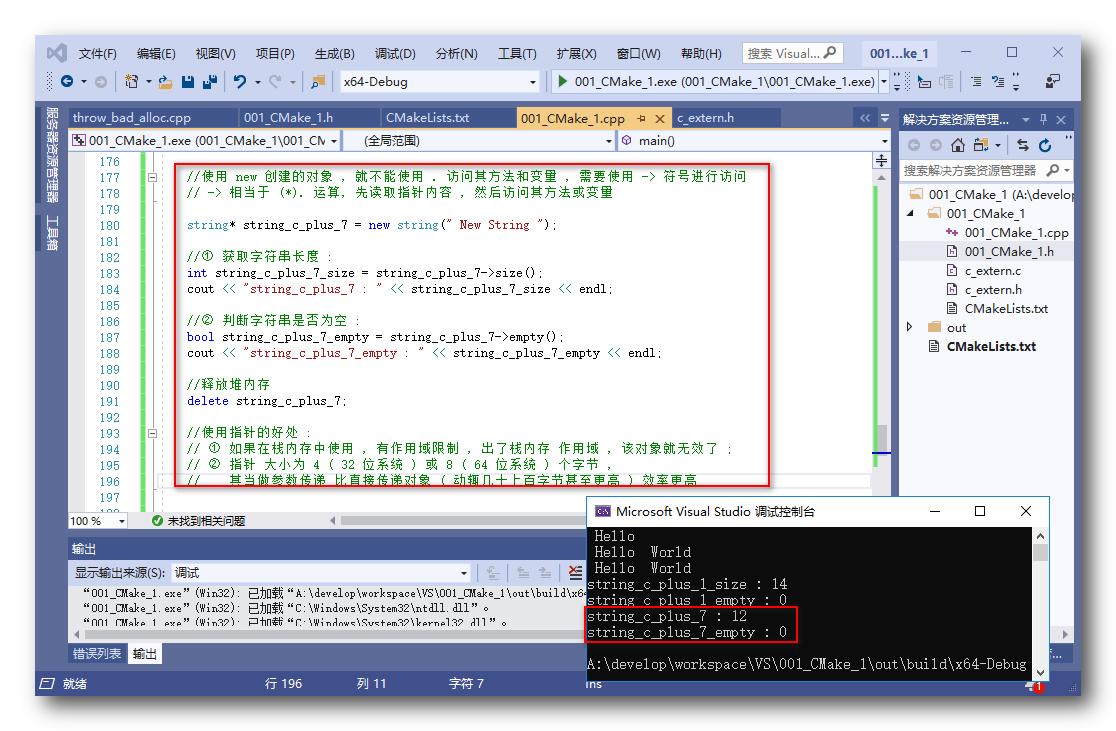C / C++ 字符串 完整代码示例

// 001_CMake_1.cpp: 定义应用程序的入口点。
//

#include "001_CMake_1.h"
#include "c_extern.h"

using namespace std;

//定义方法接收 int& 引用类型变量
//并在方法中修改该变量的值
void quote(int& b) {
b = 888;
}

int main()
{
cout << "Hello CMake。" << endl;

//1. C C++ 兼容
//博客地址 : https://hanshuliang.blog.csdn.net/article/details/98840708
//调用 c_extern.h 头文件中定义的方法
//该方法定义在了 C 语言文件中

//2. 引用数据类型
//博客地址 : https://hanshuliang.blog.csdn.net/article/details/99239635
//代码 展示 流程 :
//① 定义 普通 类型 变量
int a = 8;
//② 定义 引用类型变量, 格式 : 类型名称& 变量名 = 对应类型变量名称 ;
int& b = a;
//③ 调用函数传入引用类型参数 : 将引用类型传给接收引用类型的方法
quote(b);
//④ 打印引用数据类型的修改结果 , 结果是 b 被修改成了 888
cout << b << endl;

//引用数据类型定义与使用 :
// ① 引用数据类型定义 : 类型名称& 变量名 = 对应类型变量名称 ;
// ② 引用数据类型的使用方法 : 直接当做原来的变量使用即可, 可以替换原来变量的位置使用

//引用类型解析 :
// ① int& 是引用数据类型 , b 是 a 的引用
// ② 分配一块内存存放 int 类型数据 8 , 将该内存赋予一个别名 a
// ③ 同时又给该内存赋予另外一个别名 b

//3. 字符串使用
//C 字符串 表示方法 :
// ① 字符数组 : 本质是 字符 数组 char[] , 这里注意字符数组要以 NULL 或 '\0' 结尾;
char string_c[] = { 'h', 'e', 'l', 'l', 'o', '\0' };
// ② 指针 : 使用指针形式表示字符串 , 默认末尾增加 '\0' ;
char* string_c_p = "hello";

//字符串打印 :
// ① 打印字符串 , cout 后的 << 后可以打印 字符串 , 也可以打印变量
// ② 输出 cout << 字符串或变量1 << 字符串或变量2 ... << endl 可以拼接 输出信息
cout << "string_c : " << string_c << endl;
cout << "string_c_p : " << string_c_p << endl;

//C 语言中的字符串操作
//拷贝字符串
char string_c_copy_destination;
char string_c_copy_source[] = { 'h', 'e', 'l', 'l', 'o', '\0' };
// ① 参数 : strcpy 方法是拷贝字符串的方法 , 第一个参数是目标字符串 , 第二个参数是源字符串
// ② 作用 : 该方法是将 源字符串 拷贝到 目标字符串中
strcpy(string_c_copy_destination, string_c_copy_source);
// ③ 打印拷贝结果 :
cout << "string_c_copy_destination : " << string_c_copy_destination << endl;

//拼接字符串
//① 定义目标字符串 : 拼接字符串的目标字符串的大小一定要大于等于要拼接的两个字符串大小之和, 否则会报错
char string_c_cat_destination = " cat dst ";
char string_c_cat_source[] = " cat src ";
//② 拼接字符串方法参数 : 第一个参数是目标字符串 , 第二个参数是源字符串
//③ 目标字符串大小 : 注意 目标字符串的 大小一定要大于 两个字符串实际大小
strcat(string_c_cat_destination, string_c_cat_source);
//④ 打印字符串拼接结果 :
cout << "string_c_cat_destination : " << string_c_cat_destination << endl;

//获取字符串长度
//① 参数 : 传入要获取的字符串 , 该长度不含 '\0' 结尾标志
//② 作用 : 获取实际的字符串长度 , 即自动识别 '\0' 位置 , 获取其长度 , 与所占用的内存大小无关
char string_c_len[] = { 'h', 'e', 'l', 'l', 'o', '\0' };
char string_c_len2 = { 'h', 'e', 'l', 'l', 'o', '\0' };
char * string_c_len3 = "hello";

//① 字符数组长度 , 测量从开始到 '\0' 的长度, 不包括 '\0'
int len1 = strlen(string_c_len);
//② 指定大小的字符数组长度 , 结果不是指定的大小的值 , 获取的是实际字符串长度
int len2 = strlen(string_c_len2);
//③ 指针表示的字符串 , 其获取的大小是实际字符串大小, 不包含自动添加的 '\0'
int len3 = strlen(string_c_len3);
//④ 打印 三个字符串大小
cout << "len1 : " << len1
<< " len2 : " << len2
<< " len3 : " << len3
<< endl;

//字符串比较
// ① 参数说明 : 参数是需要比较的两个字符串 , 第一个参数 str1 , 第二个参数 str2
// ② 对比规则 : str1 和 str2 两个字符串 , 从左到右 逐个对比 ASCII 码 大小 ;
//		a. 如果 str1 等于 str2 , 返回 0;
//		b. 如果 str1 > str2 , 返回值 大于 0 ;
//		c. 如果 str1 < str2 , 返回值 小于 0 ;

//定义需要比较的字符串
char* string_c_comp_1 = "Hello";
char* string_c_comp_2 = "Hello";
char* string_c_comp_3 = "hello";

// ① 两个字符串相等
int cmp_result_1_2 = strcmp(string_c_comp_1, string_c_comp_2);
// ② "Hello" 字符串 (H 对应 ASCII 72) 小于 "hello" 字符串 (h 对应 ASCII 104) , 返回值 小于 0
int cmp_result_1_3 = strcmp(string_c_comp_1, string_c_comp_3);
// ③ "hello" 字符串 (h 对应 ASCII 104) 大于 "Hello" 字符串 (H 对应 ASCII 72) , 返回值 大于 0
int cmp_result_3_1 = strcmp(string_c_comp_3, string_c_comp_1);

//输出字符串对比结果
cout << "cmp_result_1_2 : " << cmp_result_1_2
<< " cmp_result_1_3 : " << cmp_result_1_3
<< " cmp_result_3_1 : " << cmp_result_3_1
<< endl;

// C++ string 类 : 该类定义在 iostream 头文件中
//创建 string 类型对象有三种方法 :
//① 直接使用字符串赋值
//② 调用构造方法赋值
//③ 最后可以调用 new 为字符串分配一块内存

//① 使用字符串赋值
string string_c_plus_1 = " Hello ";

//② 调用构造方法 初始化字符串
string string_c_plus_2(string_c_plus_1);
string string_c_plus_3(" World ");

//上面的三种字符串不需要释放 , 因为其定义在栈内存中 , 下面使用 new 创建字符串的情况需要 delete 释放内存 ;

//③ 使用 new 申请的内存 , 需要使用 delete 释放
string *string_c_plus_4 = new string(" New ");
delete string_c_plus_4;

//使用 new [] 申请的数组 , 需要使用 delete[] 释放
//使用 malloc 申请的内存 , 需要使用 free 释放

//C++ 字符串输出
//字符串对象不能直接在 cout 中输出, cout << string string_c_plus_5 << endl; 是错误的
//cout << string_c_plus_2 << endl;
//要将 string 对象打印到控制台上, 需要将其转为 C 字符串 , char* 或 char[] 才能输出
cout << string_c_plus_1.c_str() << endl;

//C++ 字符串拼接
//① "+" : 操作符重载 , 重新定义了 加号运算符的行为 , 这里加号可以实现字符串拼接 , 与 Java 类似
//② 调用 string 对象的 append 方法 , 拼接字符串
string string_c_plus_5 = string_c_plus_1 + string_c_plus_3;
string string_c_plus_6 = string_c_plus_1.append( string_c_plus_3 );

//输出拼接的字符串
cout << string_c_plus_5.c_str() << endl;
cout << string_c_plus_6.c_str() << endl;

//获取 C++ 字符串长度 : 调用 string 对象的 size() 方法 , 获取字符串长度
int string_c_plus_1_size = string_c_plus_1.size();
cout << "string_c_plus_1_size : " << string_c_plus_1_size << endl;

//判断 C++ 字符串是否为空 : 调用 string 对象的 empty() 方法 ;
bool string_c_plus_1_empty = string_c_plus_1.empty();
cout << "string_c_plus_1_empty : " << string_c_plus_1_empty << endl;

//使用 new 创建的对象 , 就不能使用 . 访问其方法和变量 , 需要使用 -> 符号进行访问
// -> 相当于 (*). 运算, 先读取指针内容 , 然后访问其方法或变量

string* string_c_plus_7 = new string(" New String ");

//① 获取字符串长度 :
int string_c_plus_7_size = string_c_plus_7->size();
cout << "string_c_plus_7 : " << string_c_plus_7_size << endl;

//② 判断字符串是否为空 :
bool string_c_plus_7_empty = string_c_plus_7->empty();
cout << "string_c_plus_7_empty : " << string_c_plus_7_empty << endl;

//释放堆内存
delete string_c_plus_7;

//使用指针的好处 :
// ① 如果在栈内存中使用 , 有作用域限制 , 出了栈内存 作用域 , 该对象就无效了 ;
// ② 指针 大小为 4 ( 32 位系统 ) 或 8 ( 64 位系统 ) 个字节 ,
//	  其当做参数传递 比直接传递对象 ( 动辄几十上百字节甚至更高 ) 效率更高

return 0;
}


展开全文string
• 字符串的索引从 0 开始，这意味着第一个字符索引值为 ,第二个为 , 以此类推。 你可以在字符串中使用引号，字符串中的引号不要与字符串的引号相同: 你也可以在字符串添加转义字符来使用引号： var x = 'It\'...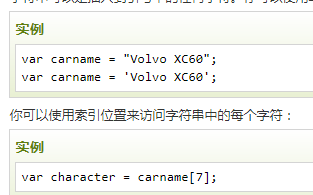字符串的索引从 0 开始，这意味着第一个字符索引值为 ,第二个为 , 以此类推。
你可以在字符串中使用引号，字符串中的引号不要与字符串的引号相同:
你也可以在字符串添加转义字符来使用引号：
var x = 'It\'s alright'; var y = "He is called \"Johnny\"";
可以使用内置属性 length 来计算字符串的长度：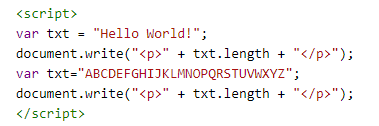字符串可以是对象
通常， JavaScript 字符串是原始值，可以使用字符创建： var firstName = "John"
但我们也可以使用 new 关键字将字符串定义为一个对象： var firstName = new String("John")
实例
var x = "John"; var y = new String("John"); typeof x // 返回 String typeof y // 返回 Object
常用函数
var x = "JohnJohn";              // x 是字符串
y = x.charAt(2); // h
y = x.charCodeAt(2); // 104
y = x.concat(y, y); // JohnJohn104104, x+y+y
y = x.indexOf('h'); // 2, 索引从0开始
y = x.lastIndexOf('h'); // 6
y = x.slice();
y = x.split('o'); //J,hnJ,hn
y = x.substr(2); // hnJohn
y = x.substring(2,4) // hn，[2,3]
y = x.toLocaleLowerCase(); // johnjohn,小写
y = x.toLocaleUpperCase(); // JOHNJOHN,大写
y = x.toString(); // 转成Stirng
y = x.toUpperCase(); // JOHNJOHN,大写
y = x.trim(); // JohnJohn,去除两端的空格
y = x.valueOf(); // 返回某个字符串对象的原始值
展开全文• 1两种数据类型 java中有两种数据类型...字符串属于常量，但却有点特殊，它属于引用数据类型，本文目的是探究学习java中关于 字符串对象的拷贝所存在的疑问，记录于此以加强记忆。 另外，对象是否相等的判断也因为...java 引用类型
• '''5.3.1 字符串对象方法''' '''在很多字符串处理和脚本应用中，内建的字符串方法是足够的。例如，一个逗号分隔的字符串可以使用split方法拆分成多块：''' val = 'a,b, guido' # print(val.split(',')) #['a', 'b', ...Pandas
• 如何获取数组的长度？如何获得集合的长度？如何获取字符串对象的长度？数组中只有length属性，所以说应该调用length属性。集合中要获取长度只有调用size方法字符串对象的长度只有调用length方法...
• 字符串对象&gt;.toUpperCase()；    返回把原字符串所有小写字母都变成大写的字符串。   下面看一下示例：   &lt;html&gt; &lt;head&gt; &lt;title&gt;String字符串对象...JavaScript HTML
• 第四讲的第二部分：在C/C++本地代码中访问Java的String字符串对象 Java与C++中字符串的区别 在Java中，使用的字符串String 对象时Unicode(UTF-16)码，即每个字符不论是中文还是英文还是符号，一个字符总是占两个...jni java c++
• 第三章-字符串对象  字符串对象定义： typedef struct { PyObject_VAR_HEAD long ob_shash; int ob_sstate; char ob_sval; } PyStringObject; 由于字符串是变长对象，所以有变长对象头。 ob_shash...python string struct list
• 首先写一个工具类JsonUtils.java... //把list转换成字符串 String cacheString = JsonUtils.objectToJson(list);JsonUtils.java工具类public class JsonUtils { // 定义jackson对象 private static final Objec...java转换
• 字符串对象 基本包装类型 为了方便操作基本数据类型，JavaScript 还提供了三个特殊的引用类型：String、Number和 Boolean。 基本包装类型就是把简单数据类型包装成为复杂数据类型，这样基本数据类型就有了属性和方法...
• 1、如果我们需要实现一个配置管理的功能，那么为每个配置项目增加一个字段既复杂也不利于扩展，所以我们通常使用一个字符串来保存配置项目信息，这里介绍如何使用json的字符串解析来达到刚才说的目的。引入Json需要...java json
• 非常多的时候我们得到的可能是对象字符串形式，这时候你可能会想到eval： 定义和用法 eval() 函数可计算某个字符串，并执行其中的的 JavaScript 代码。 语法 eval(string) ...
• 西城旧梦梦旧人 2017-05-08 21:17 前段时间讲了synchronized锁方法，锁this对象，锁非this对象。具体可以看往期的文章，今天我们... 再声明一个一样的字符串的时候，取值是从原地址去取的，也就是说是同一个对象。这
• 最初遇到的这个小问题是，如何判断一个字符串是否在一个字符串数组里面？ private static boolean isBelongList(String str){ String[] strArray = {"1","2","3"}; boolean bResult = false; for (String...Java
• 两个对象，要理解这个，就要知道string类的工作原理。下面来慢慢分析一下： public class StringTest { public static void main(String[] args){ String s1=&quot;Hello&quot;; String s2=&quot;...
• jquery：字符串转json对象，json对象字符串 JSON字符串: var str = '{ "name": "cxh", "sex": "man" }'; JSON对象: var str = { "name": "cxh", "sex": "man" }; 字符串转json对象： var str = '{ "name...
• 对象字符串 var str = JSON.stringify(Obj); obj 替换成你要转的对象 字符串对象 var str1 = JSON.parse(str); str 替换成你要转的字符串
• 对象转json字符串 string jsStr=Newtonsoft.Json.JsonConvert.SerializeObject(对象) json字符串对象 User user=JsonConvert.DeserializeObject<User>("json字符串");c#
• JSON.stringify() 对象转成字符串 JSON.parse(); 字符串转成对象
• C#对象转json字符串,C#json字符串对象,C#DataTable对象转json字符串,C#把集合转成DataTableC#json操作
• 字符串转json对象 方法一：varjson = eval('('+ str +')'); 方法二：returnJSON.parse(str); json对象字符串 JSON.stringify(jsonobj); //可以将json对象转换成字符串 例如： JSON字符串: var str1 = '{...
• object转化为json字符串 var data = new Object(); var jsonData = JSON.stringify(data); json字符串转为js对象 var jsonObj = eval(jsonStr);js
• 时间字符串转换成date对象 var new_date_str = '2017-07-07'.replace(/-/g, '/') var newdate = new Date(new_date_str) console.log(newdate)//可以输出对应的日期对象，注意传入字符串也可以是'2017-7-7' var ...javascript date
• url 对象字符串编码传参 let info = encodeURI(JSON.stringify(this.data.info)); wx.navigateTo({ url: '/pages/partner_reward/recognition_result/result?info=' + info, }) url 字符串对象解码接...传参 url编码 url解码...